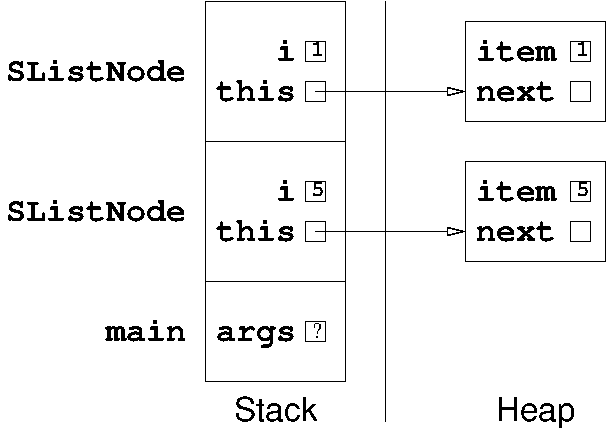CS 61B: Data Structures (Spring 2014)
Midterm I

### Solutions

Problem 1. (10 points) A miscellany.

a. We'll accept any of the following.

• To reference the object the currently running method was called on.
• To call a constructor from another constructor of the same class (operating on the same object).
• To refer to an instance variable that is overshadowed by a local variable.

b.

```
for (int i = 1; i < n; i = i + 2) {
process(n);
}
```

c.

• a: float or double.
• b: Java will only compile this code if b has type Object; however, we will also accept “X or any superclass of X.”
• c: boolean.
• d: Any reference (Object or any subclass of Object).
• e: Any superclass or subclass of Y.

d.Problem 2. (8 points) Inheritance.

```
public interface X {
public void whatever();
}

public abstract class Profile implements X {
protected double weight;              // Maximize my privacy, please.

public Profile(double weight) {
this.weight = weight;
}

public abstract double date(double food);

public int date(int food) {
return (int) date((double) food);
}

public String introduce() {
return "I'm neurotic and vindictive and I weigh " + weight;
}

public static void main(String[] args) {
Profile p = new DatingProfile(260.0);
((DatingProfile) p).diet(p.weight, 25.0);
System.out.println(p.introduce());
}
}

public class DatingProfile extends Profile {
public DatingProfile(double weight) {
super(weight - 70.0);
}

public double date(double food) {
weight = weight + (double) food;
return weight;
}

public void diet(double weight, double loss) {
weight = weight - loss;
}

public String introduce() {
return "I'm feisty and spontaneous and I weigh " + (weight - 60);
}

public void whatever() { }
}
```
The code prints: I'm feisty and spontaneous and I weigh 130.0

Problem 3. (7 points) Lengthening runs.

Here's an example of a recursive solution.

```
public int lengthenRuns() {
if (next == null) {
next = new SListNode(item, null);
return 1;
}

if (item == next.item) {
return next.lengthenRuns();
}

next = new SListNode(item, next);
return 1 + next.next.lengthenRuns();
}
```
Most students wrote iterative solutions (as opposed to recursive). This has the big advantage that you can process much longer lists without running out of stack space, but the code is somewhat more complicated.
```
public int lengthenRuns() {
int count = 1;
SListNode n = this;

while (n.next != null) {
if (n.item != n.next.item) {
n.next = new SListNode(n.item, n.next);
n = n.next;
count++;
}
n = n.next;
}

n.next = new SListNode(n.item, null);
return count;
}
```
One final reminder to the students who took the exam: In Java, this can never be null. Many of you started with comparisons like if (this == null), which are usually harmless but are always completely unnecessary.
Mail inquiries to cs61b@cory.eecs